Checkout JEE MAINS 2022 Question Paper Analysis : Checkout JEE MAINS 2022 Question Paper Analysis :

# Ohm’s Law and Resistance

Ohm’s law is one of the most popular and important laws that help us define the relationship between voltage, current and resistance. The law was first established by a German physicist named Georg Simon Ohm and was the most important theory that described the quantitative characteristics of the physics of electricity.

Ohm’s law can be also be considered as an empirical law. It can be used in developing conclusions or in reasoning while conducting many experiments especially in showing that current for some materials is approximately proportional to the electric field. We shall learn more about this law including its definition, formulas, applications and more in this lesson.

## What is Ohm’s Law?

Ohm’s law states that the current through a conductor between two points is directly proportional to the voltage across the two points.

Ohm’s law formula is written as;

V ∝ I

Therefore, V = RI where R is a constant called resistance. R depends on the dimensions of the conductor and also on the material of the conductor. Its SI unit is Ohm (Ω).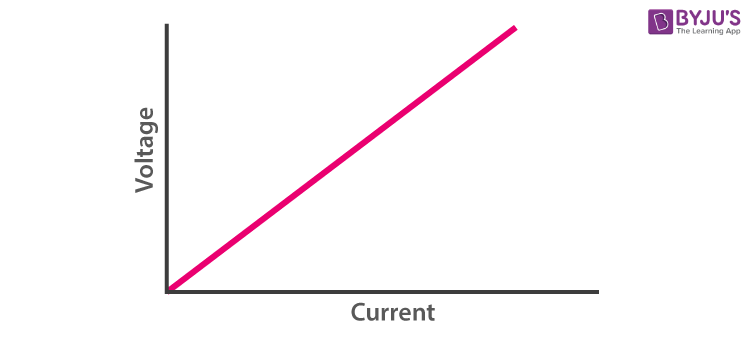Ohm’s law was found out by various experiments, somewhat similar to the thermodynamic laws. As far as its significance is concerned, this law is used withing all branches of electronic studies or science. The law is useful in carrying out calculations such as in determining the value of resistors or the current in a circuit or in measuring the voltage.

Additionally, Ohm’s law helps us describe how current flows through materials such as electrical wires, etc.

## Ohm’s Law Formulas

There are basically three types of Ohm’s law formulas or equations. They are;

• I = V / R
• V = IR
• R = V / I

Here, I is the resistance, V is voltage and R is resistance.

## Ohm’s Law Triangle

Ohm’s law is often used in the study of electronics and electricity. Therefore, it is crucial for students to remember the formulas as it will help in circuit analysis. Ohm’s law formulas given above can be easily remembered using the Ohm’s law triangle. This triangle helps us to easily represent the interchangeability of the equations. We can take a triangle and divide it into three parts. Then we can input the values, V, I, R into the triangle. V on top, I on the left side and R on the right side. It will look like this;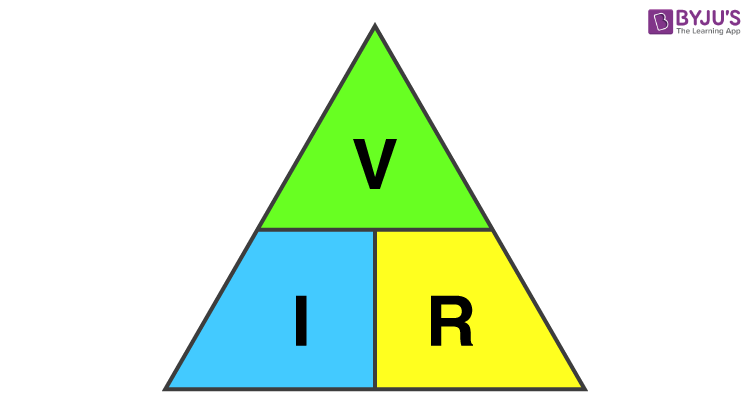Whenever you want to solve the calculation you can just cover the value you are looking for.

### Vector Form of Ohm’s Law

The vector form of Ohm’s law is used in electromagnetics and material science. The vector form is given as,

$$\begin{array}{l}\bar{J} = \sigma \bar{E}\end{array}$$

Where,

represents vector

$$\begin{array}{l}\bar{J}\end{array}$$
is the current density,
$$\begin{array}{l}\bar{E}\end{array}$$
is the electric field and σ is conductivity.

Conductivity is the reciprocal of resistivity. Which brings us to the next question, what is resistivity?

## Resistivity

Consider a conductor with a cross-sectional area ‘a’ and length ‘l’. Let its resistance be R. Let it have a voltage difference of V volts between its end and a current I flowing through it.

Now consider another conductor of the same dimensions, except its length is doubled. This can be considered as two conductors of the first kind connected in series. Therefore, their voltages add up. The voltage here will, therefore, become 2V.

From Ohm’s law, R = (V/T)

Here, R = (2V / I) = 2R

That means that resistance increased with length. That is R ∝ l

Concept point: This does make sense if you think about it. If the length increases then the flowing electrons will face more obstacles and hence resistance increases.

Similarly, now consider a conductor with half the cross-sectional area as the first conductor. This can be considered as one of the two conductors in a parallel connection. Therefore, the current becomes half of the original value I/2. Thus, the value of the current becomes I/2 now.

Here, R = (V/ (I/2)) = 2R

This means that resistance increases when the area of cross-section decreases. That is,

R ∝ 1/A

Also Read: Dimensional Formula of Resistance

Concept point: As the area of cross-section decreases the flowing electrons come into more contact with positive nuclei of metals. Thus resistance increases.

Combining these two results we get,

R ∝ l/A

Or, R = ρl / A where ρ is a constant called the coefficient of resistance or resistivity.

In the above equation if L = 1m and A = 1m2, then R = ρ.

This signifies that resistivity is the resistance of a conductor with 1m length and 1m2 area. The resistivity of a conductor is dependent on the nature of its material and certain external factors. Such as heat.

### Vector Expression

V = IR

⇒ V = I ρL/A

⇒ V = L ρI/A

⇒ V = JρL since current density J = I/A

⇒ El = JρL since V = El

Cancelling L from both sides, E = Jρ or J = E/ρ

1/ρ = σ which is conductivity.

Therefore, = σ where the bar letters are vectors.

Concept point: You might be wondering why current is a scalar unit while current density is a vector unit. To understand this you need to remember how a vector is defined. A vector is a quantity with both magnitude and direction and that obeys the laws of vector addition. The current does not follow the rules of vector addition and hence, it is not a vector.

The broader aspect of this concept that you need to understand here is that vector quantities are concepts we use to aid our calculations. So sometimes we have to make exceptions in order to make our calculations easier or even correct in some cases.

## Ohm’s law Limitations

There are some limitations to Ohm’s law. They are as follows:

• Ohm’s law is an empirical law which is found true for maximum experiments but not for all.
• Some materials are non-ohmic under a weak electric field.
• Ohm’s law holds true only for a conductor at a constant temperature. Resistivity changes with temperature.

Joules heat is given by H = I2Rt where I is current, R is resistance and t is time. As long as the current flows, greater will be the temperature of the conductor.

• Ohm’s law is not applicable to in-network circuits.
• Ohm’s does not apply directly to capacitor circuits and Inductor circuits.
• The V-I characteristics of diodes are much different.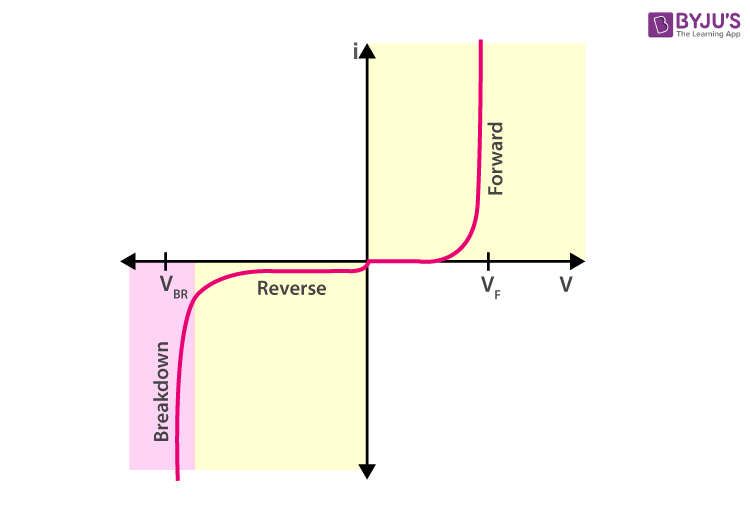• V-I graph of ohmic conductors is not really a straight graph. It does show some variation.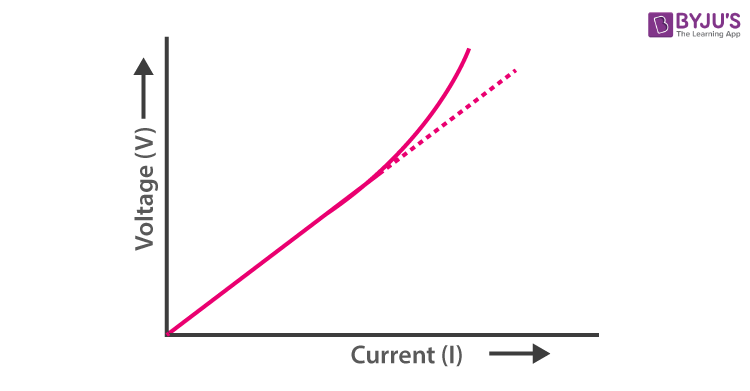## Applications of Ohm’s Law

Ohm’s law is very useful and has several applications. Here are some.

• It is widely used in circuit analysis.
• It is used in ammeter, multimeter etc.
• It is used to design resistors.
• It is used to get the desired circuit drop in circuit design.
• Advanced laws such as Kirchhoff’s Norton’s law, Thevenin’s law are based on ohm’s law.
• Electric heaters, kettles and other types of equipment working principle follow ohm’s law.
• A laptop and mobile charger using DC power supply in operation and working principle of DC power supply depend on ohm’s law.

Ohm’s law also has many other uses as well.

## Analogies of Ohm’s Law

Some analogies of ohm’s law:

### Hydraulic Analogy

A hydraulic circuit can be compared to an ohmic conductor to make the problem easier to solve. Here, pressure works similar to voltage and flow rate works similar to current.

### Temperature Analogy

Similarly, a temperature circuit can also be compared to an ohmic conductor. Here, temperature gradient works similar to voltage and heat flow works similar to current.

In fact, GS ohm discovered Ohm’s law by drawing an analogy from Fourier’s work on heat flow.

## Related Terms: Current, Voltage and Resistance

Apart from the standard definitions, you need to know what these terms actually mean. Let’s take a look at the current first. Electric current to be more precise. What comes to your mind when you first hear the word current? Perhaps a river that is flowing. That’s what electric current is, it can be visualized as the flow of electrons from one place to another.

Now, why does the flow occur? Well, a river flows from the mountains to the sea, i.e from a high place to a low place. Well, similarly current flows from a high potential to a low potential and this change of potential is called voltage.

You may notice that every river has some obstructions and that’s what resistance is to current. Resistance is the property of the conductor that obstructs the flow of current.

## Ohm’s Law Problems With Solutions

1. We have an unknown resistor which dissipates 30 watts power while dropping 15 volts across it. Find the current that is flowing through it.

Solution:

We apply Ohm’s law formula for I,

I = P/V = 30/15 = 2 A

2.  Apply ohm’s law for the following circuits?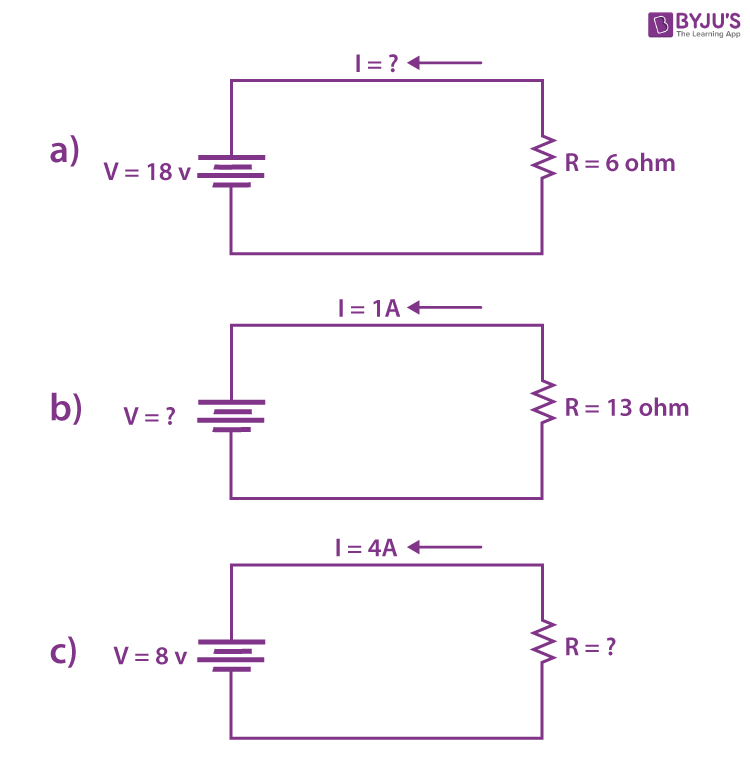Solution:

Appling ohm’s law on the circuit (a)

$$\begin{array}{l}I = \frac{V}{R}\end{array}$$

$$\begin{array}{l}I = \frac{18}{6}\end{array}$$

I = 3 A

Appling ohm’s law on the circuit (b)

V = I × R

V = 1 × 13

V = 13 V

Appling ohm’s law on the circuit (c)

$$\begin{array}{l}R = \frac{V}{I}\end{array}$$

$$\begin{array}{l}R = \frac{8}{4}\end{array}$$

R = 2 Ω

3. How much voltage will be dropped across a 50 kΩ resistance whose current is 300 µA?

Given

R = 50 kΩ = 50 × 103Ω

I = 300 µA = 300 × 10-6 A

Solution:

V = I × R

V = (300 × 10-6) × (50 × 103)

V = 15 V

4. Apply the power (P) formula for the following circuit?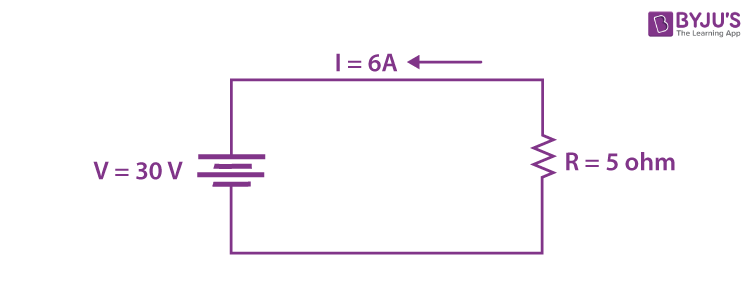Given:

I = 6 A

V = 30 V

R = 5 Ω

Solution:

Power formula: P = VI or P = I2R or

$$\begin{array}{l}P = \frac{V^{2}}{R}\end{array}$$

P = 30 × 6

P = 180 W

5. An electric circuit has a current of 3.00 A flowing through it. Find the potential difference across a resistor, with a resistance of 250 Ω.

Solution:

Using the Ohm’s Law formula:

V = IR = (3.00 A)(250 Ω)

V = 750V

The potential difference across the resistor in the circuit is 750 V.

## Electrical Resistance and Ohm’s Law – Video Lesson## Frequently Asked Questions on Ohm’s law and Resistance

### How does the resistance of the conductor vary with its length?

The resistance of a conductor is directly proportional to its length.

### State Ohm’s law?

The current through the conductor is directly proportional to the potential difference between its ends, provided the temperature and the other physical conditions remain the same.

### Define Ohm?

The resistance will be equal to 1 ohm if a current of 1 ampere flows through it under a potential difference of 1 volt.

### What is the SI unit of resistance?

The SI unit of resistance is Ohm.

### What is the dimensional formula for resistance?

The dimensional formula for resistance is given by [M1L2T-3I-2].

Test your Knowledge on Ohms law and resistance